# RS Aggarwal Solutions for Class 8 Maths Chapter 19 Three-Dimensional Figures

RS Aggarwal Solutions For Class 8 Maths Chapter 19 Three-Dimensional Figures are provided here. You can download the pdf of RS Aggarwal Solutions for Class 8 Maths Chapter 19 Three-Dimensional Figures from the given links. Class 8 is an important phase of a student’s life. It is critical to thoroughly understand the concepts taught in Class 8 as they are continued in Class 9 and 10, while also building a foundation. Here we will learn about the Three-Dimensional Figures, their properties.

RS Aggarwal Solutions help students to get a good score in the examinations by providing extensive knowledge about the subject, as Class 8 is a critical stage in their academic career. Therefore, we at BYJU’S provide answers to all questions uniquely and briefly.

## Download pdf of RS Aggarwal Solutions For Class 8 Maths Chapter 19 Three-Dimensional Figures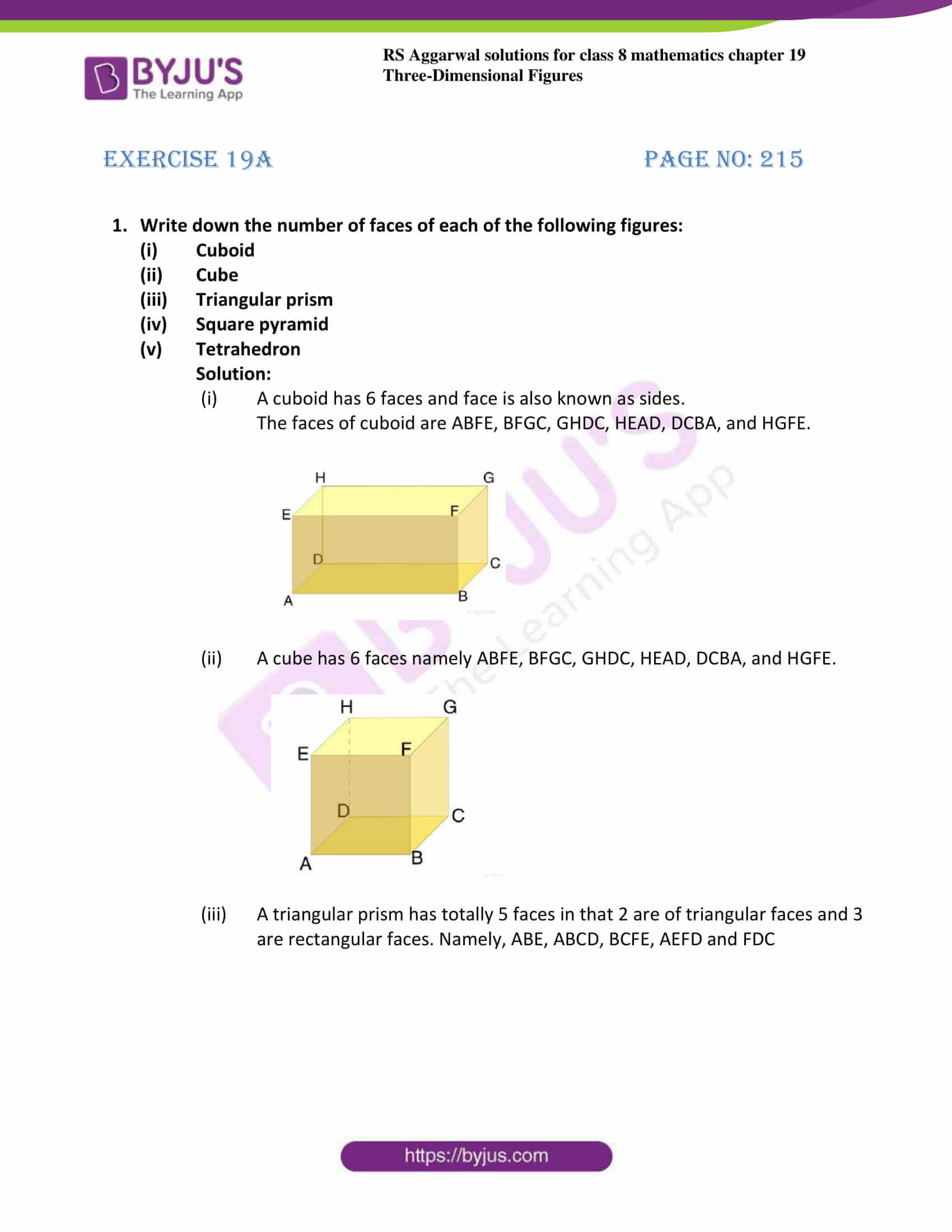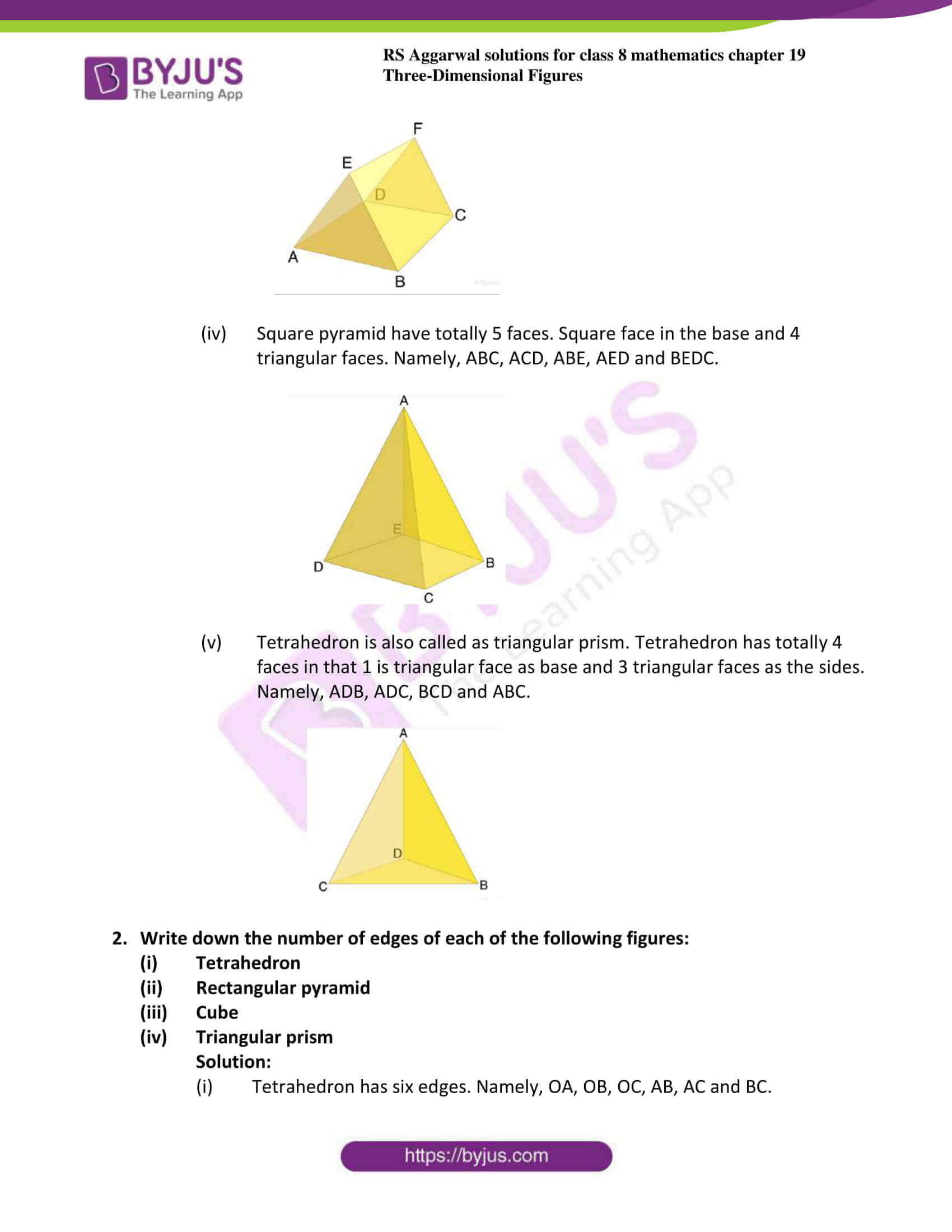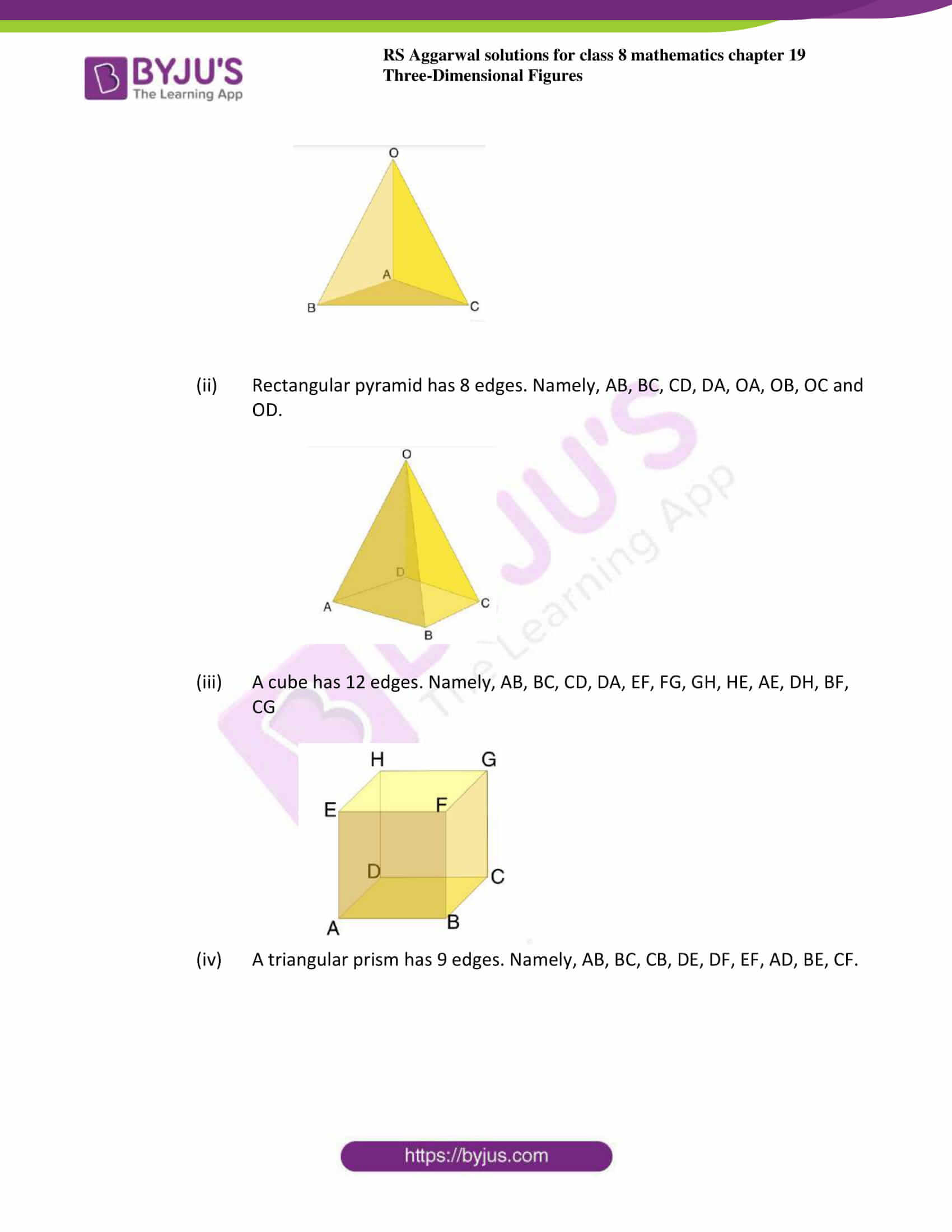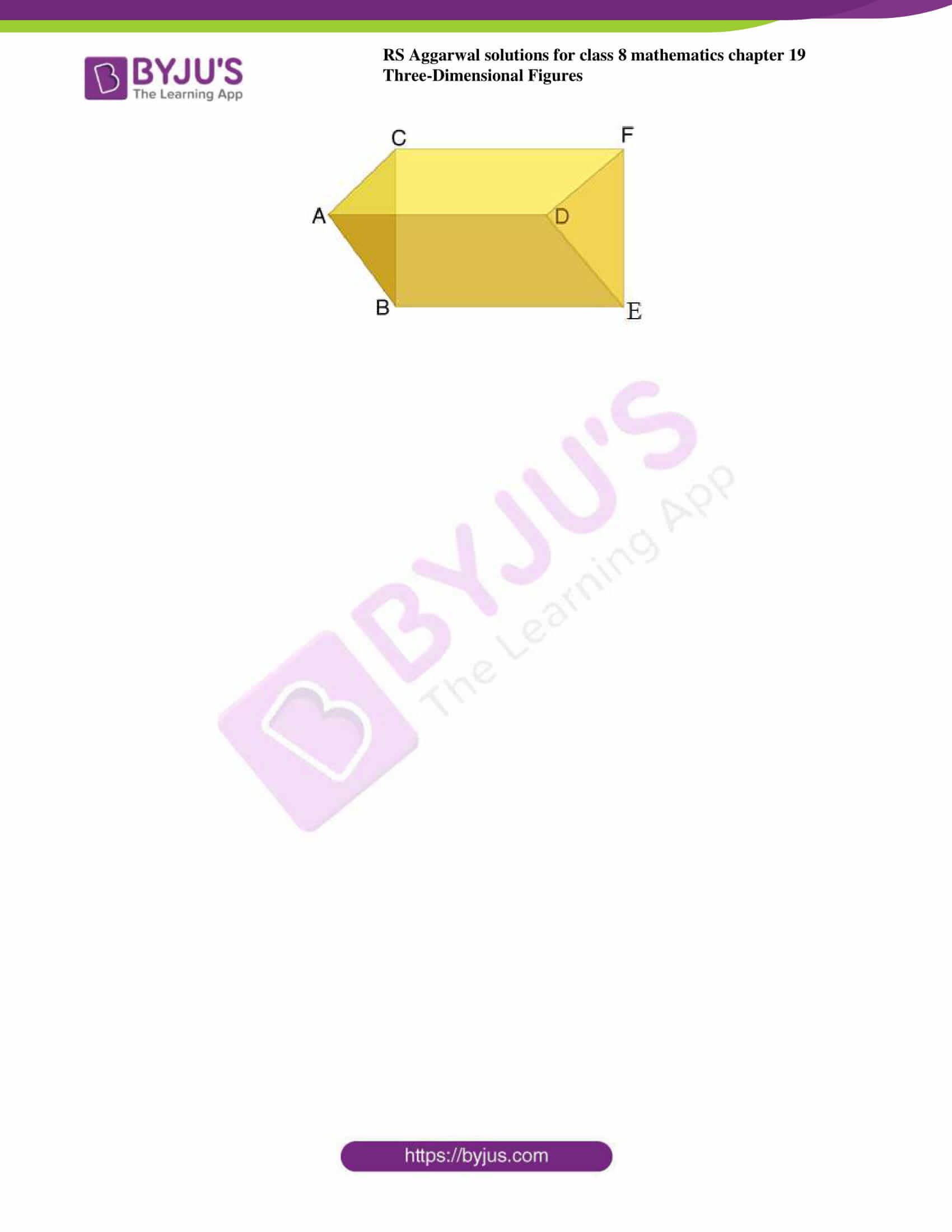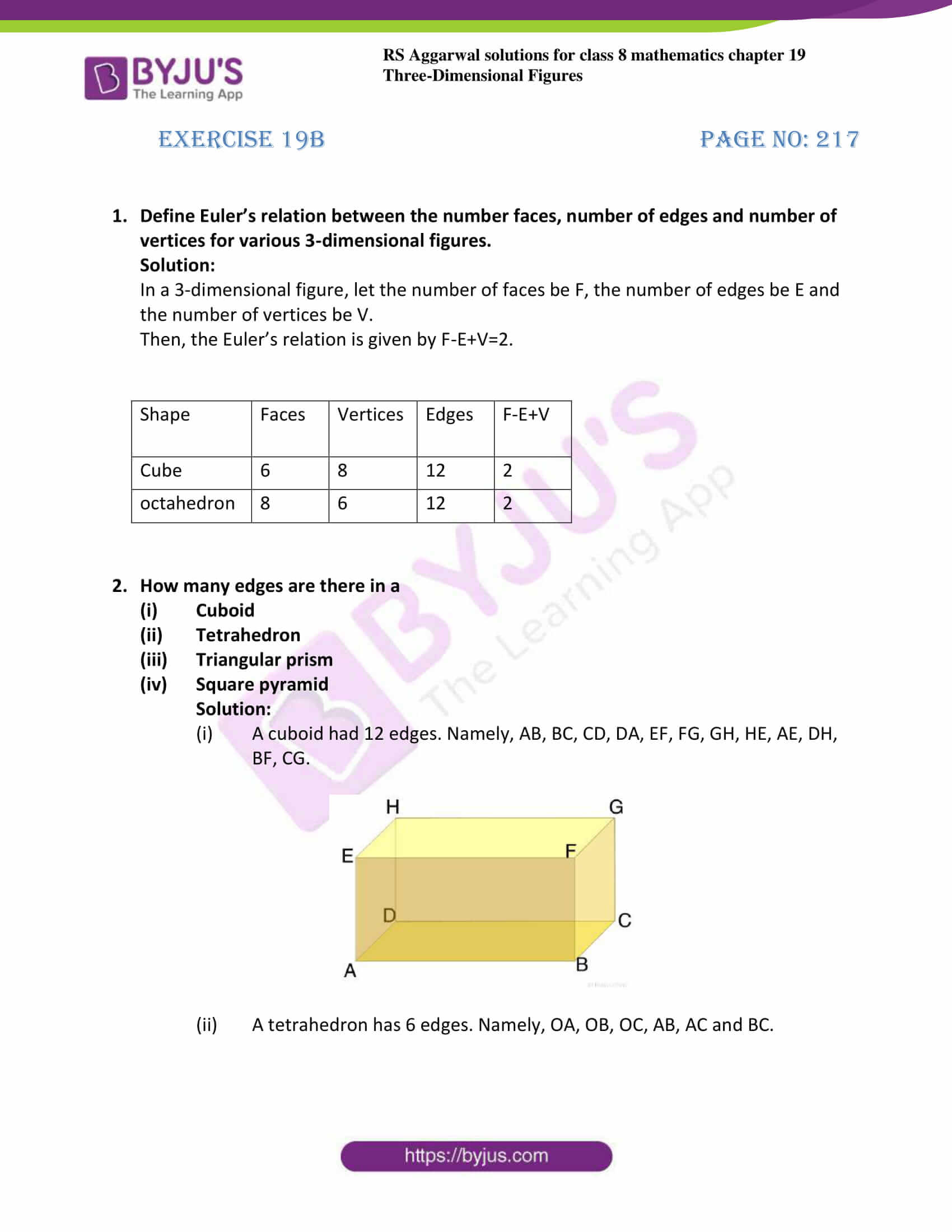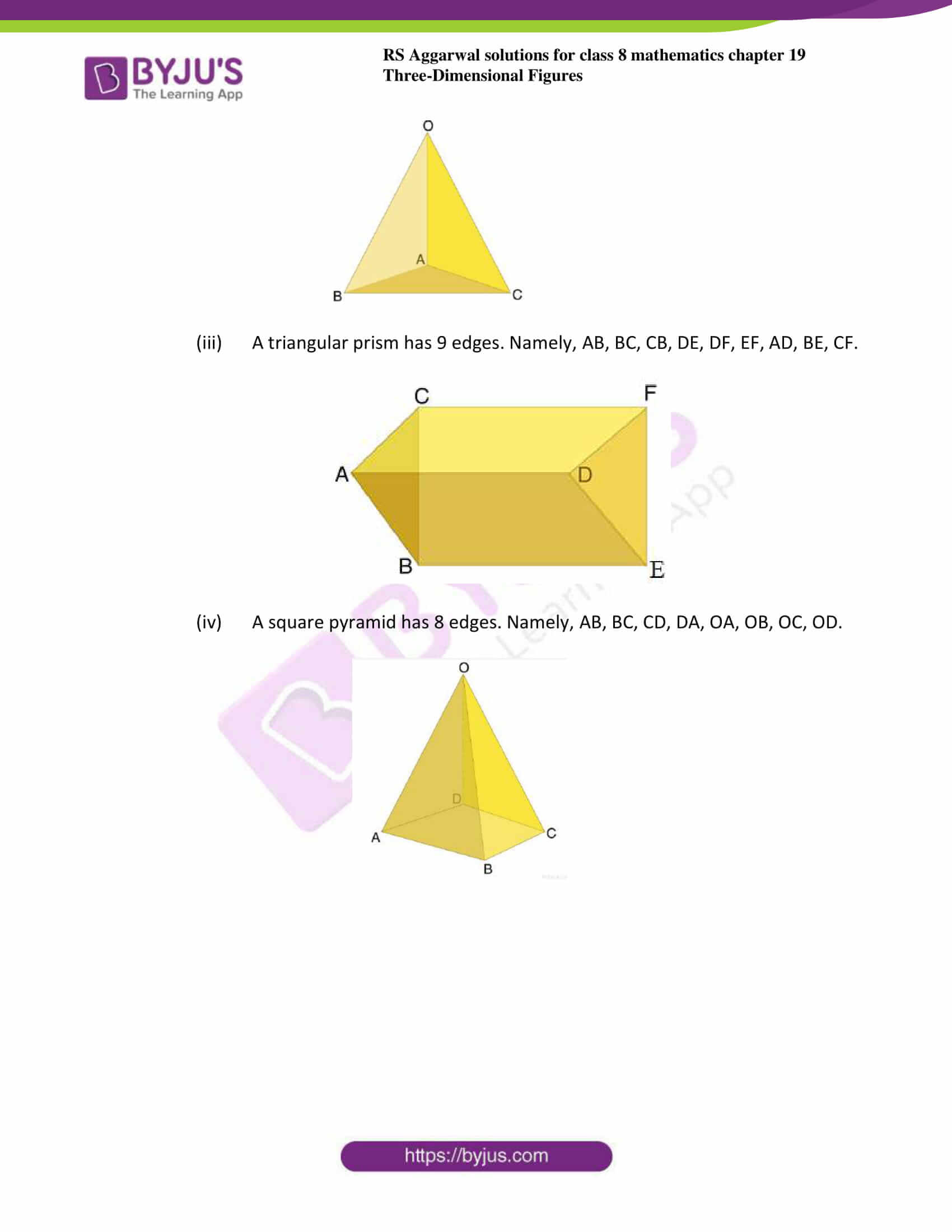## Exercise 19A

1. Write down the number of faces of each of the following figures:

1. Cuboid
2. Cube
3. Triangular prism
4. Square pyramid
5. Tetrahedron

Solution:

1. A cuboid has 6 faces and face is also known as sides.The faces of cuboid are ABFE, BFGC, GHDC, HEAD, DCBA, and HGFE.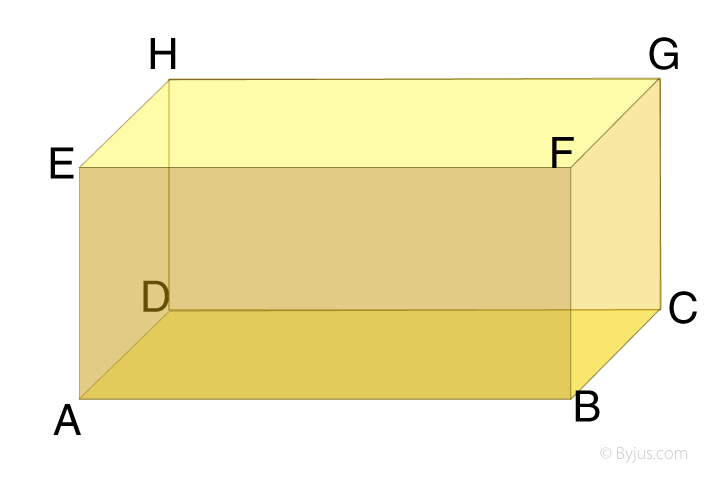2. A cube has 6 faces namely ABFE, BFGC, GHDC, HEAD, DCBA, and HGFE.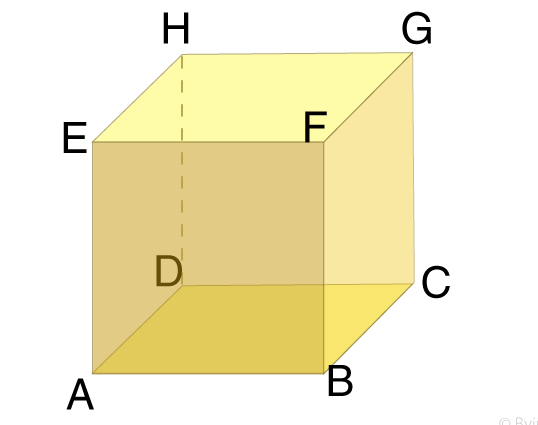3. A triangular prism has totally 5 faces in that 2 are of triangular faces and 3 are rectangular faces. Namely, ABE, ABCD, BCFE, AEFD and FDC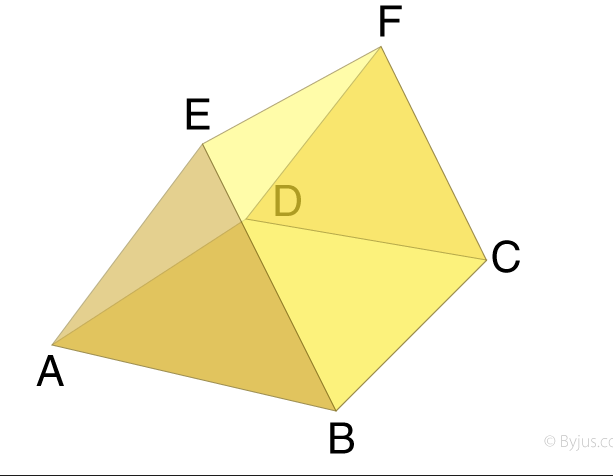4. Square pyramid have totally 5 faces. Square face in the base and 4 triangular faces. Namely, ABC, ACD, ABE, AED and BEDC.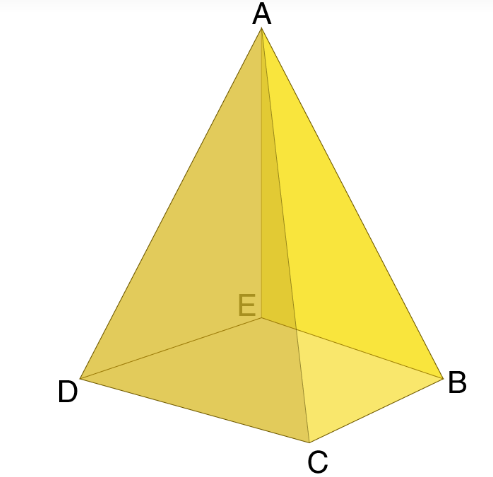(v) Tetrahedron is also called as triangular prism. Tetrahedron have totally 4 faces in that 1 is triangular face as base and 3 triangular faces as the sides. Namely, ADB, ADC, BCD and ABC.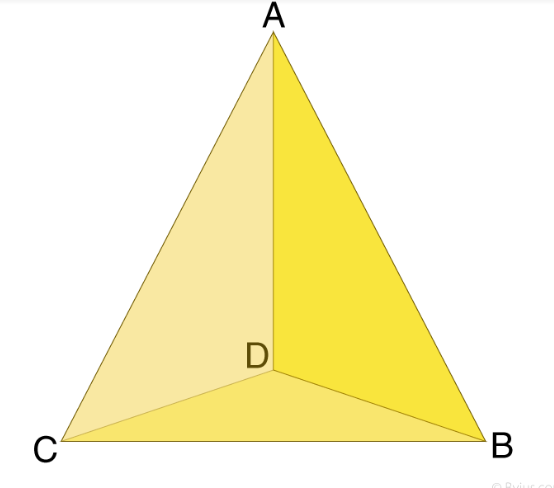2. Write down the number of edges of each of the following figures:

1. Tetrahedron
2. Rectangular pyramid
3. Cube
4. Triangular prism

Solution:

1. Tetrahedron has six edges. Namely, OA, OB, OC, AB, AC and BC.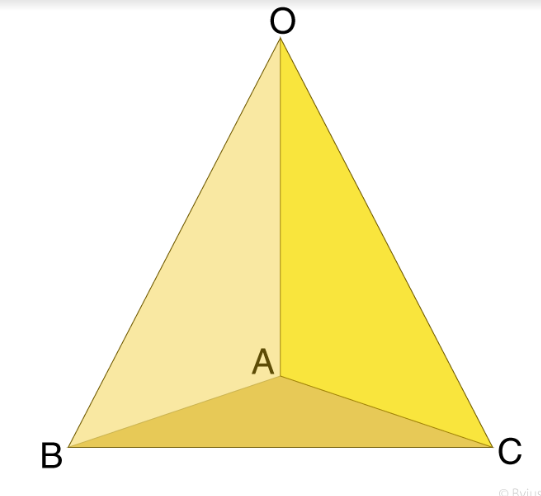2. Rectangular pyramid has 8 edges. Namely, AB, BC, CD, DA, OA, OB, OC and OD.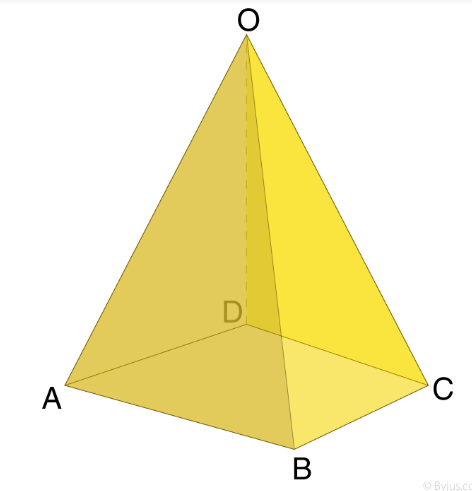3. A cube has 12 edges. Namely, AB, BC, CD, DA, EF, FG, GH, HE, AE, DH, BF, CG.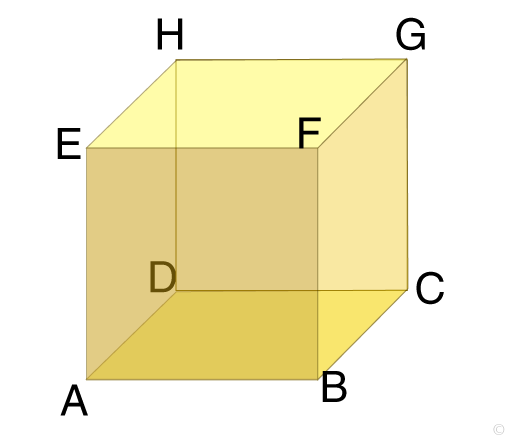4. A triangular prism has 9 edges. Namely, AB, BC, CB, DE, DF, EF, AD, BE, CF.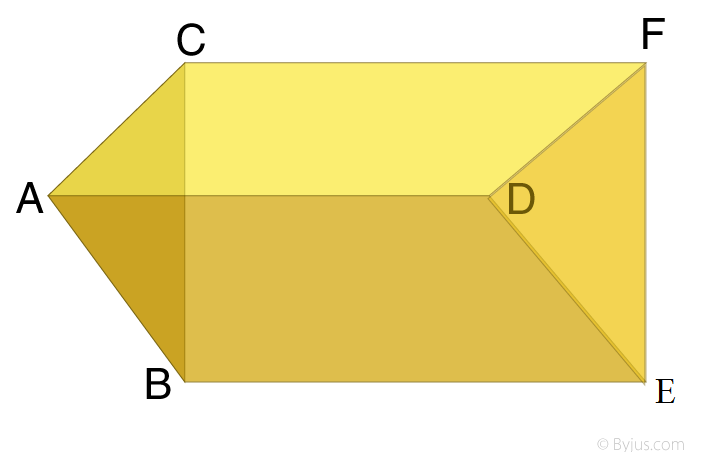## Exercise 19B

1. Define Euler’s relation between the number faces, number of edges and number of vertices for various 3-dimensional figures.

Solution:

In a 3-dimensional figure, let the number of faces be F, the number of edges be E and the number of vertices be V.

Then, the Euler’s relation is given by F-E+V=2.

 Shape Faces Vertices Edges F-E+V Cube 6 8 12 2 octahedron 8 6 12 2

2. How many edges are there in a

1. Cuboid
2. Tetrahedron
3. Triangular prism
4. Square pyramid

Solution:

1. A cuboid had 12 edges. Namely, AB, BC, CD, DA, EF, FG, GH, HE, AE, DH, BF, CG.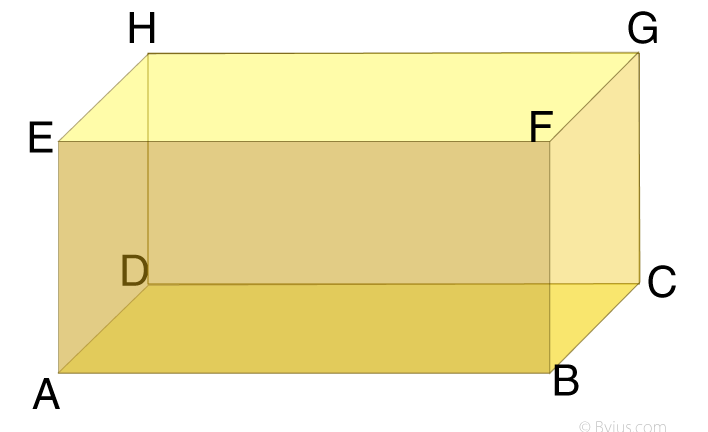2. A tetrahedron has 6 edges. Namely, OA, OB, OC, AB, AC and BC.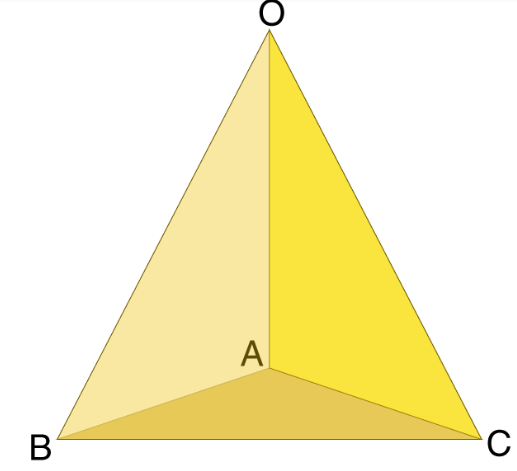3. A triangular prism has 9 edges. Namely, AB, BC, CB, DE, DF, EF, AD, BE, CF.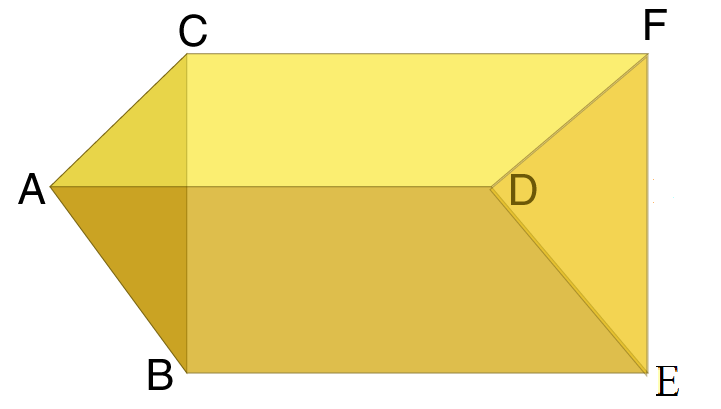4. A square pyramid has 8 edges. Namely, AB, BC, CD, DA, OA, OB, OC, OD.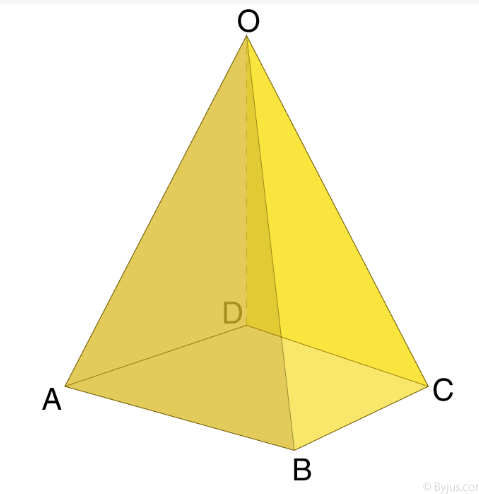## RS Aggarwal Solutions for Class 8 Maths Chapter 19- Three-Dimensional Figures

Chapter 19, Three-Dimensional Figures, contains 2 Exercises. RS Aggarwal Solutions given here contains the answers to all the questions present in these exercises. Let us have a look at some of the concepts that are being discussed in this Chapter.

• Definition of solids
• Faces, vertices and edges of a three-dimensional figures
• Cuboid
• Cube
• Prism
• Pyramid
• Square pyramid
• Rectangular pyramid
• Triangular pyramid
• Eulers’s relation for Three-Dimensional Figures

Exercise 19A

Exercise 19B

### Chapter Brief of RS Aggarwal Solutions for Class 8 Maths Chapter 19 – Three-Dimensional figures

The RS Aggarwal Solutions for Class 8 Maths Chapter 19 – Three-Dimensional Figures deals with the definition of solids, faces, vertices and edges of a Three-Dimensional Figures such as cuboid, cube, etc, and also deals with Euler’s relation for Three-Dimensional Figures.